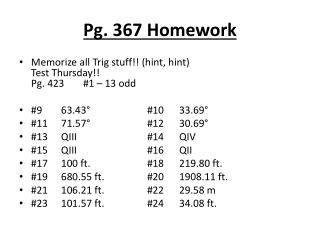DownloadDownload PresentationPg. 367 Homework

Pg. 367 Homework

Télécharger la présentationPg. 367 Homework

- - - - - - - - - - - - - - - - - - - - - - - - - - - E N D - - - - - - - - - - - - - - - - - - - - - - - - - - -
Presentation Transcript

1. Pg. 367 Homework • Memorize all Trig stuff!! (hint, hint)Test Thursday!!Pg. 423 #1 – 13 odd • #9 63.43° #10 33.69° • #11 71.57° #12 30.69° • #13 QIII #14 QIV • #15 QIII #16 QII • #17 100 ft. #18 219.80 ft. • #19 680.55 ft. #20 1908.11 ft. • #21 106.21 ft. #22 29.58 m • #23 101.57 ft. #24 34.08 ft.

2. 6.6 Applying Trig Functions • A boat travels at 35 mph for 2 hr on a course 53° east of north; then it changes to a course 143° east of north and travels for another 3 hrs. Determine the distance from the boat to its home port. What is the bearing from the boat’s home port to its location at the end of 5 hr?

3. 7.3 Solving Trig Equations and Inequalities Graphically Solving Trig Functions Find all solutions to the equation2cos2x + sin x + 1 = 0 Find all solutions to the equation3sin3 (2x) – 2cos x = 0 • Find all solutions of cosx = 0.8 • Find all solutions to the equation tan x = 3cos x

4. 7.3 Solving Trig Equations and Inequalities Graphically Solving Trig Inequalities How many solutions are there to the equation • Solve sin x < cot x • Find all solutions tosin (2x) ≥ x2 – 1

5. 7.3 Solving Trig Equations and Inequalities Analytically Solving a Trig Equation by Factoring Find all solutions totan x = 3cos x over the interval [0, 2π] Find all solutions in one period of the equation3tan4x = 1 + sec2x • Find all solutions to2cos2x + sin x + 1 = 0 in the interval [0, 2π] • Find the solutions to(cosx – 1)(sin x + 0.7) = 0in the interval [0, 2π]